# Everything about RSI - page 144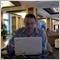Moderator
121441

Forum on trading, automated trading systems and testing trading strategies

How to Start with Metatrader 5

Sergey Golubev, 2020.04.15 10:14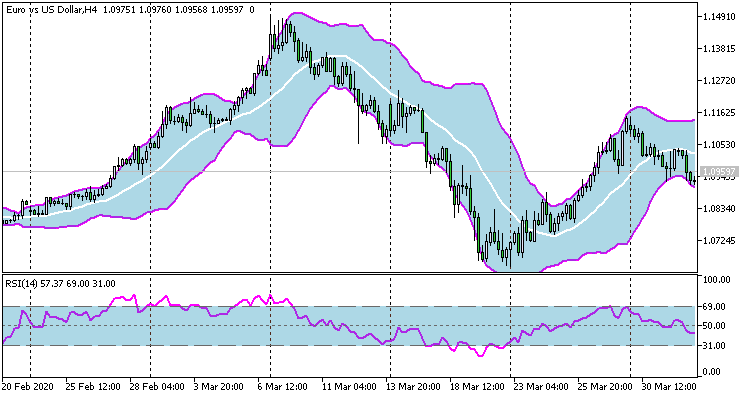Dual Candle-stick Strategy thread
The strategy is related to Bollinger Bands Fills and RSI Fills indicators with template file.
It is going to be converted to the EA I hope.

for information.

Forum on trading, automated trading systems and testing trading strategies

Dual Candle-stick Strategy

Vladimir Karputov, 2020.04.19 07:42

I published a Strategy Advisor: Dual Candlestick Strategy EA:

In the description, I marked some points with gray color - these are the nuances that I noticed during testing, but have not yet included in version 1. Before starting the adviser, I recommend that you FULLY read the entire description (use the built-in translator: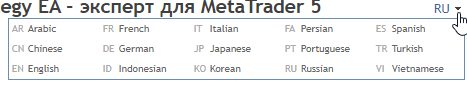)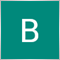10

I am after composite RSI MT5 that is based on the formula like one below. I am not sure how i an search this 144 page of RSI topic. As this one include both MT4 and 5, difficult to scan all the code.
Composite RSI is:

RSI2 + RSI9 values for 4H, Daily, Weekly timeframes then divided by 6 to give the average.
It can be found on the top right corner over here (https://www.forexfactory.com/attachm...7&d=1587149526)Moderator
121441

RSI Level Breakout - indicator for MetaTrader 5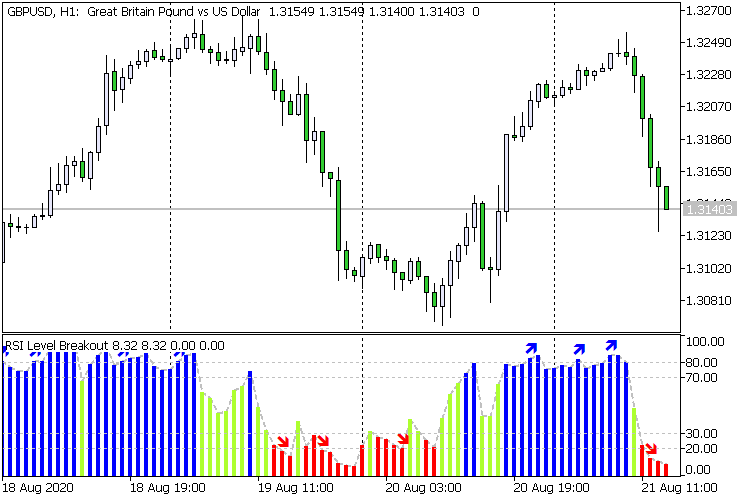The indicator is focused on trend continuation signals when levels are broken.7

Hello Sirs,

Is it possible to have this "RSI cyclic smoothed v2" to be coded in mq4 or ex4?

(ps. From "TradingView")

```// Copyright (C) 2017 CC BY, whentotrade / Lars von Thienen
// Source:
// Book: Decoding The Hidden Market Rhythm - Part 1: Dynamic Cycles (2017)
// Chapter 4: "Fine-tuning technical indicators for more details on the cRSI Indicator
//
// Usage:
// You need to derive the dominant cycle as input parameter for the cycle length as described in chapter 4.
//
// License:
// This work is licensed under a Creative Commons Attribution 4.0 International License.
// You are free to share the material in any medium or format and remix, transform, and build upon the material for any purpose,
// even commercially. You must give appropriate credit to the authors book and website, provide a link to the license, and indicate
// if changes were made. You may do so in any reasonable manner, but not in any way that suggests the licensor endorses you or your use.
//
study(title="RSI cyclic smoothed", shorttitle="cRSI")
src = close
domcycle = input(20, minval=10, title="Dominant Cycle Length")
crsi = 0.0
cyclelen = domcycle / 2
vibration = 10
leveling = 10.0
cyclicmemory = domcycle * 2

//set min/max ranges?

h1 = hline(30, color=color.silver, linestyle=hline.style_dashed)
h2 = hline(70, color=color.silver, linestyle=hline.style_dashed)

torque = 2.0 / (vibration + 1)
phasingLag = (vibration - 1) / 2.0

up = rma(max(change(src), 0), cyclelen)
down = rma(-min(change(src), 0), cyclelen)
rsi = down == 0 ? 100 : up == 0 ? 0 : 100 - 100 / (1 + up / down)
crsi := torque * (2 * rsi - rsi[phasingLag]) + (1 - torque) * nz(crsi)

lmax = -999999.0
lmin = 999999.0

for i = 0 to cyclicmemory - 1 by 1
if nz(crsi[i], -999999.0) > lmax
lmax := nz(crsi[i])
lmax
else
if nz(crsi[i], 999999.0) < lmin
lmin := nz(crsi[i])
lmin

mstep = (lmax - lmin) / 100
aperc = leveling / 100

db = 0.0
for steps = 0 to 100 by 1
testvalue = lmin + mstep * steps
above = 0
below = 0
for m = 0 to cyclicmemory - 1 by 1
below := below + iff(crsi[m] < testvalue, 1, 0)
below

ratio = below / cyclicmemory
if ratio >= aperc
db := testvalue
break
else
continue

ub = 0.0
for steps = 0 to 100 by 1
testvalue = lmax - mstep * steps
above = 0
for m = 0 to cyclicmemory - 1 by 1
above := above + iff(crsi[m] >= testvalue, 1, 0)
above

ratio = above / cyclicmemory
if ratio >= aperc
ub := testvalue
break
else
continue

lowband = plot(db, "LowBand", color.aqua)
highband = plot(ub, "HighBand", color.aqua)
fill(h1, h2, color=color.silver, transp=90)
fill(lowband, highband, color=color.gray, transp=90)
plot(crsi, "CRSI", color.fuchsia)```Moderator
121441

Two iRSI in one subwindow - indicator for MetaTrader 5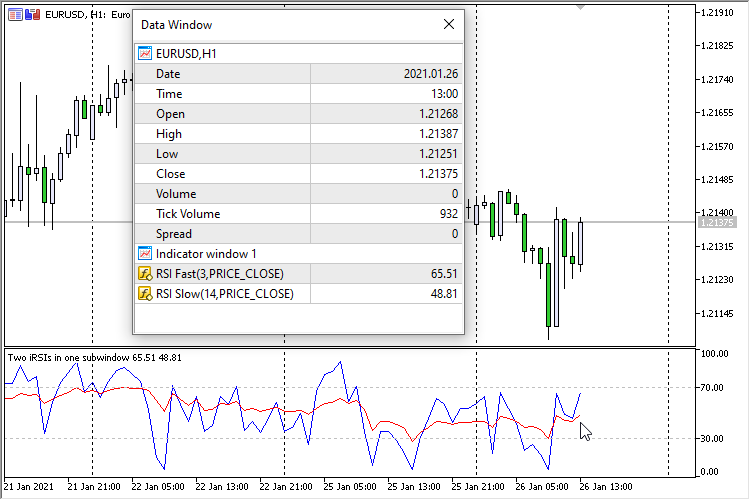Place two iRSI (Relative Strength Index, RSI) indicators - ' Fast ' and ' Slow ' in one subwindow. Moreover, each indicator has independent parameters:

• XXXX averaging period - averaging period
• XXXX type of price - price type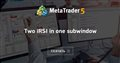Two iRSI in one subwindow
• www.mql5.com
Два индикатора iRSI (Relative Strength Index, RSI) в одном подокнеModerator
121441

RSI LiDo Color - indicator for MetaTrader 5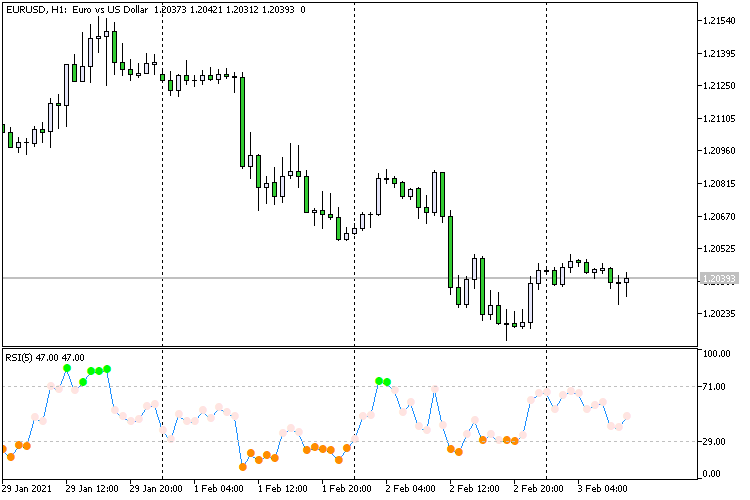The initial idea to display the iRS (Relative Strength Index, RSI) indicator as dots was in RSI LiDo .

Now the indicator has two adjustable, through input parameters, levels (' RSI: Level UP ' and ' RSI: Level DOWN ') and three colors for pointsModerator
121441

RSI Trend - indicator for MetaTrader 5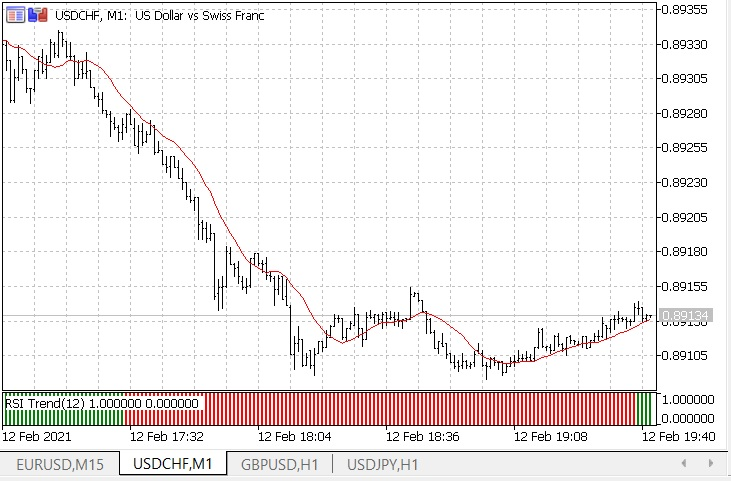As you know, oscillators have overbought and oversold zones. When moving sideways, the indicator lines do not stay in these zones for a long time. However, when the price is trending, the indicator lines are drawn in these zones for quite a long time. The peculiarity of the RSI indicator is that it reaches overbought and oversold zones much less frequently than the same Stochastic or CCI indicator, which gives fewer signals about a possible change in the direction of movement (often false).

----------------

Same indicator for MT4 is on this CodeBase page.